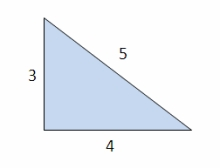# Circumference 7655

The triangle has a circumference of 35 cm. One side is four times longer than the other and, at the same time, 1 cm longer than the third. Determine the sides of the triangle.

a =  16
b =  4
c =  15

### Step-by-step explanation:

a+b+c=35
a = 4b
a = 1 + c

a+b+c=35
a = 4·b
a = 1 + c

a+b+c = 35
a-4b = 0
a-c = 1

Row 2 - Row 1 → Row 2
a+b+c = 35
-5b-c = -35
a-c = 1

Row 3 - Row 1 → Row 3
a+b+c = 35
-5b-c = -35
-b-2c = -34

Row 3 - -1/-5 · Row 2 → Row 3
a+b+c = 35
-5b-c = -35
-1.8c = -27

c = -27/-1.8 = 15
b = -35+c/-5 = -35+15/-5 = 4
a = 35-b-c = 35-4-15 = 16

a = 16
b = 4
c = 15

Our linear equations calculator calculates it.Did you find an error or inaccuracy? Feel free to write us. Thank you!

Tips for related online calculators
Do you have a linear equation or system of equations and looking for its solution? Or do you have a quadratic equation?